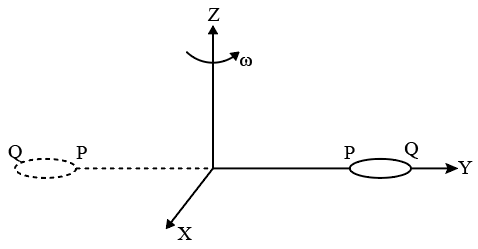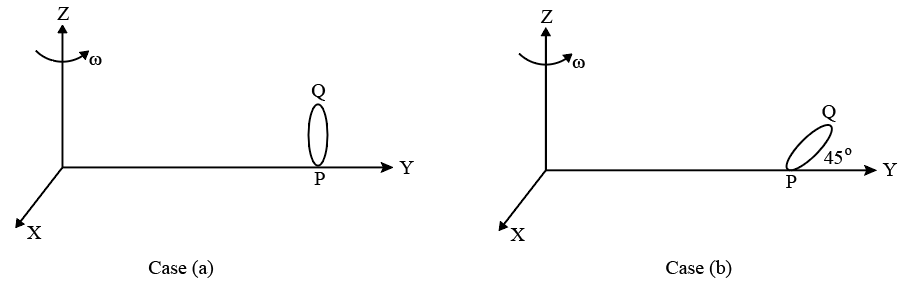Instructions

Most materials have therefractive index, n > 1. So, when a light ray from air enters a naturally occurring material, then by Snells' law, $$\frac{\sin \theta_1}{\sin \theta_2} = \frac{n_2}{n_1}$$, it is understood that the refracted ray bends towards the normal. But it never emerges on the same side of the normal as the incident ray.According to electromagnetism, the
refractive index of themedium is given by the relation, $$n = \left(\frac{c}{v}\right) = \pm \sqrt{ε_r\mu_r}$$ where c is the speed of electromagnetic waves in vacuum, v its speed in the medium, $$ε_r$$ and $$\mu_r$$ are negative, one one must choose the negative root of n. Such negative refractive index materials can now be artificially prepared and are calledmeta-materials.
They exhibit significantly different optical behavior, without violating any physical laws. Since n is negative, it results in a change in the direction of propagation of the refracted light. However, similar to normalmaterials, the frequency of light remains unchanged upon refraction even inmeta-materials.

Question 11

Question 12

# For light incident from air on a meta-material, the appropriate ray diagram is :

Instructions

The general motion of a rigid body can be considered to be a combination of (i) a motion --- centre of mass about an axis, and (ii) its motion about an instantanneous axis passing through center of mass. These axes need not be stationary. Consider, for example, a thin uniform welded (rigidly fixed) horizontally at its rim to a massless stick, as shown in the figure. Where disc-stick system is rotated about the origin ona horizontal frictionless plane with angular sp--- $$\omega$$, the motion at any instant can be taken as a combination of (i) a rotation of the centre of mass the disc about the z-axis, and (ii) a rotation of the disc through an instantaneous vertical axis pass through its centre of mass (as is seen from the changed orientation of points P and Q). Both the motions have the same angular speed $$\omega$$ in the case.Now consider two similar systems as shown in the figure: case (a) the disc with its face ver--- and parallel to x-z plane; Case (b) the disc with its face making an angle of $$45^\circ$$ with x-y plane its horizontal diameter parallel to x-axis. In both the cases, the disc is weleded at point P, and systems are rotated with constant angular speed $$\omega$$ about the z-axis.Question 13

Question 14

# Which of the following statements about the instantaneous axis (passing through the centre of mass) is correct?

Instructions

For the following questions answer them individually

Question 15

Question 16

Question 17

Question 18

Question 19

Question 20

OR